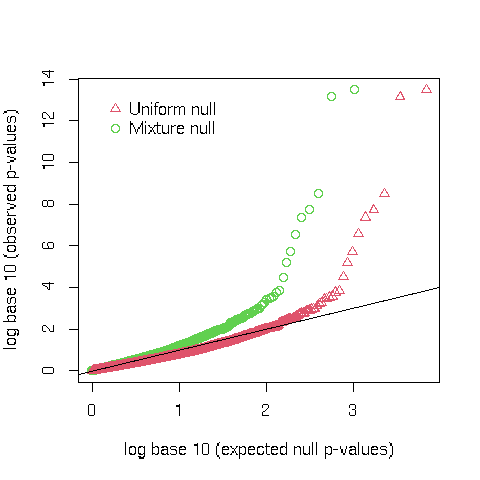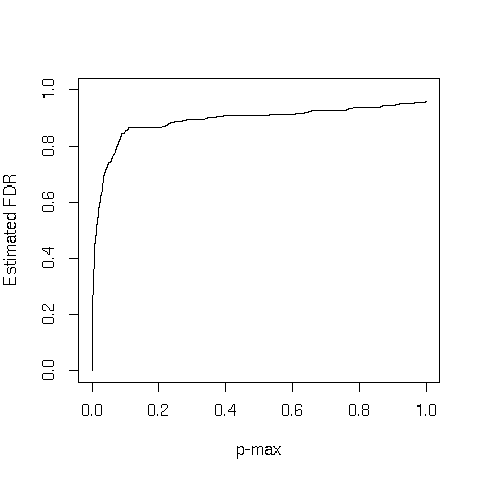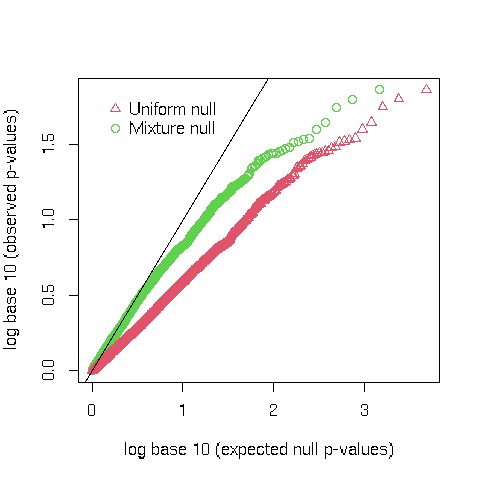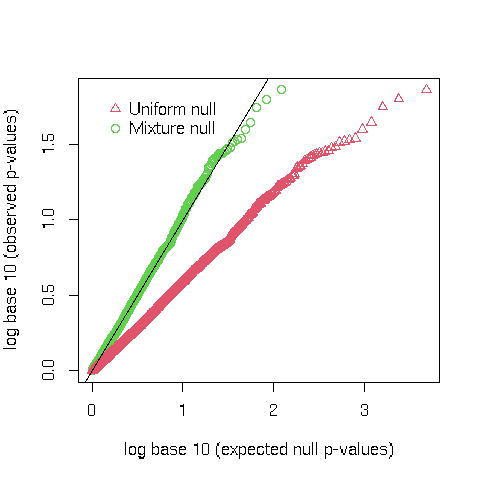# HDMT

### A Multiple Testing Procedure For High-dimensional Mediation hypotheses

Mediation analysis is of rising interest in clinical trials and epidemiology. The advance of high-throughput technologies has made it possible to interrogate molecular phenotypes such as gene expression and DNA methylation in a genome-wide fashion, some of which may act as intermediaries of treatment, external exposures and life-style risk factors in the etiological pathway to diseases or traits. When testing for mediation in high-dimensional studies like ours (Dai, Stanford, and LeBlanc 2020), properly controlling the type I error rate remains a challenge due to the composite null hypothesis.

Among existing methods, the joint significance (JS) test is an intersection-union test using the maximum p-value for testing the two parameters, though a naive significance rule based on the uniform null p-value distribution (JS-uniform) may yield an overly conservative type I error rate and therefore low power. This is particularly a concern for high-dimensional mediation hypotheses for genome-wide molecular intermediaries such as DNA methylation.

In this R package we develop a multiple-testing procedure that accurately controls the family-wise error rate (FWER) and the false discovery rate (FDR) for testing high-dimensional mediation composite null hypotheses. The core of our procedure is based on estimating the proportions of three types of component null hypotheses and deriving the corresponding mixture distribution (JS-mixture) of null p-values.

library(HDMT)

### Two Examples shown in the JASA paper

We show two examples assessing the mediation role of DNA methylation in prostate cancer studies. The first study is on assessing the mediation potential role of DNA methylation in genetic regulation of gene expression in primary prostate cancer (PCa) samples from The Cancer Genome Atlas (snp_input). The second study investigated the potential mediation of DNA methylation on the effect of exercise lowering the risk of metastatic Progression (exercise_input).

The input data (both snp_input and exercise_input) have two columns. See notation in (Dai, Stanford, and LeBlanc 2020). Column 1 contains the p-values for testing if an exposure is associated with the mediator ($$\alpha\neq0$$). Column 2 contains the p-value for testing if a mediator is associated with the outcome after adjusted for the exposure ($$\beta \neq 0$$) methylation in exercise effect on prostate cancer progression in a Seattle-based patient cohort.

data(snp_input)
dim(snp_input)
#>  69602     2
data(exercise_input)
dim(exercise_input)
#>  47900     2
##### 1. DNA Methylation in Genetic Regulation of Gene Expression Among Prostate Cancer Risk SNPs

We first read the input data matrix "input_pvalues" first. To save time for compiling this vignettes file, we use 10% of p-values in the input data matrix. To reproduce the figure in the JASA paper, one need to run the code on the entire data matrix.

input_pvalues <- snp_input
input_pvalues=input_pvalues[sample(1:nrow(input_pvalues),size=ceiling(nrow(input_pvalues)/10)),]

The first step of the procedure is to estimate the proportions of the three type of null hypotheses using the nullprop function

nullprop <- null_estimation(input_pvalues)
nullprop
#> $alpha10 #>  0.03429823 #> #>$alpha01
#>  0.4268424
#>
#> $alpha00 #>  0.4965163 #> #>$alpha1
#>  0.9233587
#>
#> $alpha2 #>  0.5308145 We next compute the expected quantiles of the mixture null distribution of $$p_{max}$$ (maximum of two p-values) using the adjust_quantile function, with either the approximation method (option exact=0) or the exact method (option exact=1). This set of quantiles can be used to draw the corrected q-q plot. pnull1<-adjust_quantile(nullprop$alpha00,nullprop$alpha01,nullprop$alpha10,nullprop$alpha1,nullprop$alpha2,input_pvalues,exact=1)

HDMT provides a function correct_qqplot to draw the corrected quantile-quantile plot for $$p_{max}$$, based on the estimated quantile of the mixture null distribution (green dots) and compared to the standard q-q plot based on the uniform distribution (red dots).

pmax <- apply(input_pvalues,1,max)
correct_qqplot(pmax, pnull1)We can also compute the pointwise estimated FDR for $$p_{max}$$ using the fdr_est() function, with either the approximation method or the exact method. The family-wise error rate cut-off can be computed by the fwer_est() function.

efdr <- fdr_est(nullprop$alpha00,nullprop$alpha01,nullprop$alpha10,nullprop$alpha1,nullprop$alpha2,input_pvalues,exact=0) plot(pmax[order(pmax)],efdr[order(pmax)],type="l",ylim=c(0,1),xlab="p-max",ylab="Estimated FDR")##### 2.DNA Methylation and Association of Physical Activity With Lower Risk of Metastatic Progression For this example, we only included 10% of p-values from the genome-wide testing as shown in the paper, due to data storage space limit. We read in the input data matrix with two columns of p-values as follows. input_pvalues <- exercise_input #To save time, we use 10% of rows input_pvalues=input_pvalues[sample(1:nrow(input_pvalues),size=ceiling(nrow(input_pvalues)/10)),] The estimation procedures are identical to the previous example: nullprop <- null_estimation(input_pvalues) We can compute the null distribution of pmax using the approximation method in Section 2.2 of the paper, and we can also compute the null distribution of $$p_{max}$$ using the exact method in Section 2.4. pnull<-adjust_quantile(nullprop$alpha00,nullprop$alpha01,nullprop$alpha10,nullprop$alpha1,nullprop$alpha2,input_pvalues,exact=0)
pnull1<-adjust_quantile(nullprop$alpha00,nullprop$alpha01,nullprop$alpha10,nullprop$alpha1,nullprop\$alpha2,input_pvalues,exact=1)

The Q-Q plot based on the approximation method is shown as follows:

pmax <- apply(input_pvalues,1,max)
correct_qqplot(pmax, pnull)The Q-Q plot based on the exact method is shown as follows:

correct_qqplot(pmax, pnull1)##### session information
sessionInfo()
#> R version 4.1.2 (2021-11-01)
#> Platform: x86_64-pc-linux-gnu (64-bit)
#> Running under: Ubuntu 18.04.4 LTS
#>
#> Matrix products: default
#> BLAS/LAPACK: /app/software/OpenBLAS/0.3.12-GCC-10.2.0/lib/libopenblas_haswellp-r0.3.12.so
#>
#> locale:
#>   LC_CTYPE=en_US.UTF-8       LC_NUMERIC=C
#>   LC_TIME=en_US.UTF-8        LC_COLLATE=C
#>   LC_MONETARY=en_US.UTF-8    LC_MESSAGES=en_US.UTF-8
#>   LC_PAPER=en_US.UTF-8       LC_NAME=C
#>  LC_MEASUREMENT=en_US.UTF-8 LC_IDENTIFICATION=C
#>
#> attached base packages:
#>  stats     graphics  grDevices utils     datasets  methods   base
#>
#> other attached packages:
#>  HDMT_1.0.5
#>
#> loaded via a namespace (and not attached):
#>   Rcpp_1.0.7       highr_0.9        plyr_1.8.6       bslib_0.3.1
#>   compiler_4.1.2   pillar_1.6.4     jquerylib_0.1.4  tools_4.1.2
#>   digest_0.6.28    jsonlite_1.7.2   evaluate_0.14    lifecycle_1.0.1
#>  tibble_3.1.5     gtable_0.3.0     pkgconfig_2.0.3  rlang_0.4.12
#>  DBI_1.1.1        yaml_2.2.1       xfun_0.27        fastmap_1.1.0
#>  stringr_1.4.0    dplyr_1.0.7      knitr_1.36       generics_0.1.1
#>  sass_0.4.0       vctrs_0.3.8      tidyselect_1.1.1 grid_4.1.2
#>  qvalue_2.26.0    glue_1.4.2       R6_2.5.1         fansi_0.5.0
#>  fdrtool_1.2.16   rmarkdown_2.11   reshape2_1.4.4   purrr_0.3.4
#>  ggplot2_3.3.5    magrittr_2.0.1   splines_4.1.2    scales_1.1.1
#>  htmltools_0.5.2  ellipsis_0.3.2   assertthat_0.2.1 colorspace_2.0-2
#>  utf8_1.2.2       stringi_1.7.5    munsell_0.5.0    crayon_1.4.2

Dai, James Y., Janet L. Stanford, and Michael LeBlanc. 2020. “A Multiple-Testing Procedure for High-Dimensional Mediation Hypotheses.” Journal of the American Statistical Association.